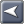﻿ ROI Computation Methodology
Captools/net Documentation

ROI Computation Methodology

 ROI Computation MethodologyROI Computation MethodologyTrue ROI for an investment is properly computed using the internal rate of return (IRR) method. This is the method used by Captools/net.  IRR takes into account both the timing and the amount of all cash flows into and out of an investment.  It is also a total return because it includes both income and capital appreciation.

On a lay person's level it is easiest to think of return on investment as being the effective Annual Percentage Rate ("APR") on an investment. The effective annual interest rate you receive on a savings account is in fact the ROI on that account.

On a more technical level, IRR is computed by converting all transactions to cash flows (positive, negative or null depending on transaction type) and solving the following equation for "Rate":

Initial Investment = Σ ( CF( i ) / (1+Rate)t(i) )

i=1 to n

where:

Initial Investment  -  The initial investment or value of the investment at the start of the ROI period.

CF(i)  - Cash Flow for transaction number "i".  Cash flow "n" is the end of period value of the investment or the final liquidating transaction for the investment.

Rate  - IRR%/100, where "IRR%" is the same as return on investment (ROI).

^t(i)  -  Time since initial investment for "i"th cash flow. (to the "t(i)" power).

Sum  - Signifies to sum all transactions starting with the first transaction following the start date specified, up to and including all transactions on the close date.

Because Rate cannot be directly solved by mathematical manipulation, it is solved iteratively.  This means that values for Rate are repetitively plugged into the equation until the equation balances.  In a few rare cases the program can fail to converge on a Rate, in which case no result (blank) is returned.

One key to properly computing internal rate of return ROI is understanding which cash flows matter. To the owner of a portfolio, the only cash flows that matter are those into and out of his/her pocket.  A cash flow out of his/her pocket and into the portfolio is a deposit.  A cash flow out of the portfolio and into his/her pocket is a withdrawal.  The value of a portfolio at the start of a period is considered to be an initial investment or deposit and thus is an out-of-pocket negative cash flow.  At the end of the ROI period, the portfolio is considered to be liquidated with funds returned to the portfolio owner and thus is equivalent to a positive or in-to-pocket cash flow.

Portfolio transactions which represent receipt of non-pocket funds or the manipulation of funds within the portfolio but which do not affect pocket cash flow do not matter.  Examples of "don't matter" transactions are dividends or interest received from portfolio security holdings, but which are retained in the portfolio.  These may be "re-invested" in cash or may be reinvested in the same, or a different security.  Although these transactions may increase portfolio value, they may also be offset by reductions in security values.  Thus they "don't matter" by themselves, what matters is the net impact on portfolio value of all non-pocket transactions.  The fact that the portfolio is assumed to be liquidated on the ROI close date captures this total net impact, and thus eliminates the need to take into account each separate non-pocket transaction explicitly.

In the case of the ROI of an individual security, the treatment is slightly different.  In this case, any buy type transaction is considered to be out-of-pocket cash flow (even if funds came from portfolio cash) and any sell, or non-reinvested dividends are considered to be in-to-pocket cash flow.  Reinvested dividends are "wash" transactions since they combine an out-of-pocket buy type transaction with an in-to-pocket dividend transaction.